# Graph clustering and community detection via Semidefinite Programming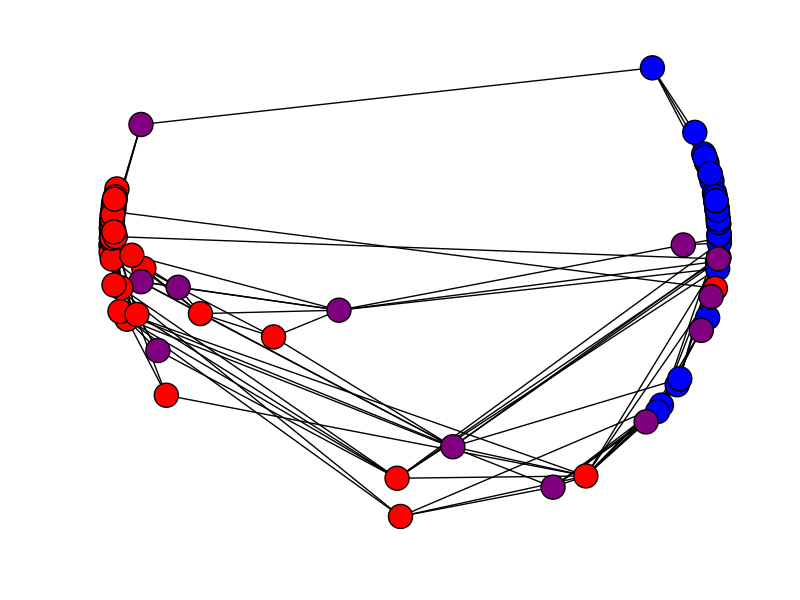Motivation: Given an undirected graph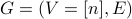, we want to partition its vertices into disjoint subsets. This task arises in applications to clustering, and network analysis. A common strategy is the following: Construct a low-dimensional embedding of the vertices. Explicitly, for each vertex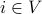, contruct a vector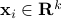, with ambient dimension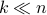. Use some greedy procedure to cluster the points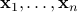. The code presented here provides an efficent way to implement step 1 by solving a semidefinite programming relaxation. On the left side, we show a small two-dimensional example. (A two-dimensional embedding of a network of political books from Mark Newman's repository. Colors correspond to political orientation. The embedding separates quite clearly two groups.)

Semidefinite Programming (SDP): Let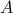denote the adjacency matrix of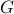, and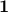the ‘all ones’ vector. We then use the following SDP: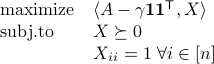Here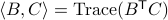denotes the usual scalar product between matrices, and we write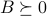if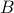is positive semidefinite.

Solving this SDP by generic methods can be quite slow. We reduce the complexity by constraining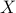to have rank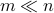, and changing variables. We introduce the ‘spin’ variables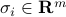and attempt to solve the following non-convex problem: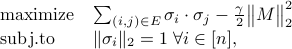where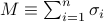. Somewhat surprisingly, we found that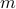as small as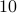to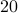is sufficient to guarantee global convergence in most practical examples (even if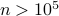).

The embedding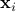is simply a low-dimensional projection of the spin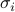(with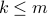).

## Paper

A. Javanmard, A. Montanari, and F. Ricci-Tersenghi, Phase Transitions in Semidefinite Relaxations, 2015

## Related Papers

S. Burer, R. D.C. Monteiro, A nonlinear programming algorithm for solving semidefinite programs via low-rank factorization, Mathematical Programming 2003

A. Montanari and S.Sen, Semidefinite Programs on Sparse Random Graphs and their Application to Community Detection, 201